# A New Controller for Voltage and Stability Improvement of Multi Machine Power System Tuned by Wind Turbine

A New Controller for Voltage and Stability Improvement of Multi Machine Power System Tuned by Wind Turbine

Issam GricheSabir Messalti  Kamel Saoudi Mohamed Yaakoub Touafek Fares Zitouni

Department of Electrical Engineering, Faculty of Technology, University of M’sila, M’sila 28000, Algeria

Department of Electrical Engineering, Faculty of Engineering, University of Bouira, Bouira 10000, Algeria

Mechatronics Laboratory (LMETR) - E1764200 Optics and Precision Mechanics Institute Ferhat Abbas University Setif 1, Setif 19000, Algeria

Sonelgaz, Transmission Network Manager, Setif 19000, Algeria

Corresponding Author Email:
issam.grich@univ-bouira.dz
Page:
81-88
|
DOI:
https://doi.org/10.18280/mmep.080110
3 August 2020
|
Accepted:
14 November 2020
|
Published:
28 February 2021
| Citation

OPEN ACCESS

Abstract:

This paper proposes a new controller for stability and voltage improvement of power networks equipped by wind turbine which optimize the dynamical response of power systems performances (voltage and transient stability) after fault. The proposed control algorithm based on new Adaptive Neuro-Fuzzy Inference System (ANFIS) controller to enhance the mechanical power of the synchronous machine into power system. The efficiency of developed control strategy has been tested using IEEE 9 Bus. Simulation results have showed that the proposed method perform better performances over wide range of disturbances for three considered scenarios studied.

Keywords:

power system, voltage improvement, wind turbine (WT), ANFIS controller

1. Introduction

Over the last few years, electric power system a have been combined with new generation connected to the power systems. As the world shifts, its emphasis from fossil fuel to clean power, researchers are desperately searching for the perfect clean energy technology. Wind, solar, wave, tidal, biofuel and biomass are all experiencing renewed interest and new research funding. One alternative clean energy technology that bears looking at is wind powers which has been used for powering transportation and industrial equipment in cities and replace the power plants [1, 2]. Wind power generation is growing rapidly in recent years around the world. The conventional point forecasting of wind power is difficult to meet the demand of power grid planning and operation [3, 4].

A dynamic and nonlinear power system in presence of wind generator one of the most complex man made system the world have seen . Small instabilities usually transpires due to changes in inertia, load conditions variations, electrical fault, voltage failure and a major change in continuous demand supply . Therefore, new controllers are able to improve the voltage and stability damping by the coordinated control based wind power generation was discussed by the authors [7-11]. From the studies, wind turbine equipped with ANFIS controller has been applicable to deal the problem of stability and voltage improvement issues with the power scheme. Among them, high penetration of wind power generation has been tested [12, 13]. The focus of recent research has been on a stability problem during integration in power system . However, very few publications can be found are available in the literature that discusses to solve the problem of stability and voltage regulation .

In the past few years, the ANFIS controller has been widely used in the wind power generation using an optimization algorithm to select reasonable parameters of the model . However, from the literatures, it shows that the optimal coordinated design of different controllers have been addressed to a single machine whereas the less attention have been paid for multi machine power system. In sum, it is clear from the literature survey that the application of different techniques to solve the problem of voltage enhancement has been established. This encourages us to propose a new technique to deal with this problem [16-18].

Recently, to satisfy the necessity of the control system and escape the rising difficulty encountered by the conventional regulators scheme, Artificial Intelligence controllers have been widely developed . A Neural Network observer of doubly fed induction generator (DFIGs) was established to increase the oscillatory stability of the power system including wind turbine . A coordination of ANFIS and type-2 fuzzy system was joined to increase a large-scale IEEE 39-bus multi-machine test system . Also, a new technique of a novel fuzzy neural PI controller based static synchronous series compensator and a fuzzy controller having a novel assembly was instantaneously established  for suppressing of low frequency oscillations on a 4-machine, IEEE test system. Another novel fuzzy rule matrix was design  for fuzzy logic system to efficiently damped low frequency oscillations of a SMIB and 4-machine, IEEE test system.

In this designed, an effective approach ANFIS controller to advance the test system voltage profile and reduce the oscillations of the power system in presence of the wind turbine connected in the bus load which strong three-phase fault is applied.

The main contributions of this work are given by the following points:

• Proposed a new ANFIS controller to improve the mechanical power required into the synchronous machines
• The effectiveness of the ANFIS controller to enhance the stability and voltage profile.
• The advantages of the proposed control approach have been validated considering the IEEE 9 Bus power system in fault disturbances.

The arrangement of this paper is presented: Section 2 describes the power system under study. Section 3 the overall framework of the proposed method which introduces the methodologies of the proposed controller. Section 4 illustrates the time domain simulation which provides the results of the proposed method and comparative studies. The conclusions are drawn in Section 5.

2. Power System Modeling under Study

Figure 1 shows the IEEE 9bus power system including 3 generators linked together by 6 lines 230 kV lines of different length which the parameters are given . It was purposely designed to review low frequency electromechanical oscillations in large interconnected power systems. Regardless of its small size, it mimics very closely the behavior of typical systems in actual operation. Each synchronous generator is equipped with rated power 247.5, 192, 128 MVA respectively. The load is represented as constant impedances. Since the surge impedance loading of a single line is about 125, 90, 100 MW located in the bus 5, 6 and 8 respectively. To study the efficient method of wind, a three wind farm are connected in 3 bus loads, bus 5, 6 and 8 which the details in ref. .

## 1.png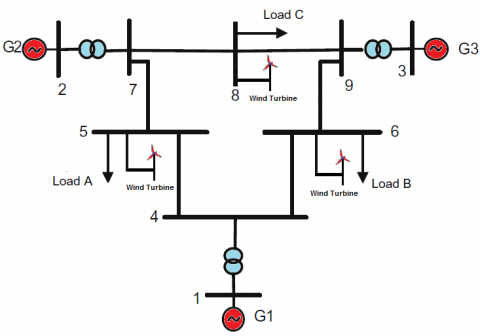Figure 1. Structure of the 9-bus power system under study

The effectiveness of the proposed controller is evaluated under multiple system operating conditions as below:

• Condition-1: without integration of wind farm and without controller;
• Condition-2: with integration of wind farm and with conventional regulator;
• Condition-3: with integration of wind farm and with ANFIS controller.

2.1 Synchronous generator model (SGM)

In this study, the dynamic behavior of machines is given by:

${{\dot{\delta }}_{i}}=\left( {{\omega }_{i}}-{{\omega }_{b}} \right)$    (1)

${{\dot{\omega }}_{i}}=\frac{{{\omega }_{b}}}{2{{H}_{i}}}\left[ {{P}_{mi}}-{{P}_{ei}}-{{D}_{i}}\,\left( {{\omega }_{i}}-{{\omega }_{b}} \right) \right]$     (2)

${{\dot{{E}'}}_{qi}}=\frac{1}{T{{'}_{d0i}}}\left[ {{V}_{ex\,i}}-E{{'}_{qi}}+\left( {{x}_{di}}-x{{'}_{di}} \right)I{{'}_{di}} \right]$      (3)

${{\dot{{E}'}}_{di}}=\frac{1}{T{{'}_{q0i}}}\left[ -E{{'}_{qi}}-\left( {{x}_{qi}}-x{{'}_{qi}} \right)I{{'}_{qi}} \right]$     (4)

$\frac{d{{V}_{ex}}}{dt}=\frac{1}{{{T}_{E}}}\left[ {{V}_{R}}-\left( {{S}_{E}}+{{K}_{E}} \right){{V}_{ex}} \right]$      (5)

$\frac{d{{V}_{1}}}{dt}=\frac{1}{{{T}_{R}}}\left( {{V}_{ref}}-{{V}_{t}}-{{V}_{1}} \right)$     (6)

$\frac{d{{V}_{2}}}{dt}=\frac{1}{{{T}_{F}}}\left( {{K}_{F}}\frac{d{{V}_{ex}}}{dt}-{{V}_{2}} \right)$    (7)

2.2 Wind turbine model

The mechanical power PW captured from a wind turbine of a blade radius (R) running in a wind stream of velocity Vw is given by :

${{P}_{W}}=\frac{1}{2}\rho \pi {{R}^{2}}{{C}_{p}}\left( \lambda ,\beta \right)\,{{V}_{w}}$      (8)

The tip speed ratio (TSR) is given by:

$\lambda =\frac{R{{\omega }_{T}}}{{{V}_{w}}}$     (9)

The power coefficient curve is approximated analytically by:

${{C}_{p}}\left( \lambda ,\beta \right)=0.5109\left( \frac{116}{X}-0.4\beta -5 \right)\exp \left( -\frac{21}{X} \right)+116\lambda$      (10)

where: $X=\frac{1}{\lambda +0.08\beta }-\frac{0.035}{1+{{\beta }^{3}}}$.

In addition the global wind power generated is given by the following equation:

${{P}_{WTotal}}=\sum\limits_{i=1}^{n}{{{P}_{W}}}$      (11)

In our case, the number of wind turbine is 21 which the total power injected is 35.28 MW.

3. Design of Proposed Controller

3.1 Conventional speed regulator

A conventional speed regulator for synchronous machine system shown in Figure 2 is used in this research .

## 2.png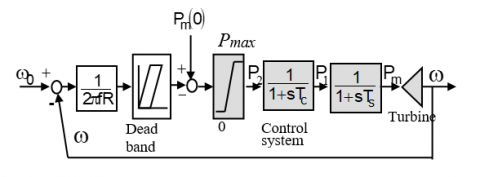Figure 2. Block diagram of governor turbine model for synchronous generator

$\frac{d{{P}_{m}}}{dt}=\frac{1}{{{T}_{s}}}\left( {{P}_{1}}-{{P}_{m}} \right)$    (12)

$\frac{d{{P}_{1}}}{dt}=\frac{1}{{{T}_{c}}}\left( {{P}_{2}}-{{P}_{1}} \right)$     (13)

${{P}_{2}}={{P}_{m}}\left( 0 \right)-\frac{1}{R}\left[ \frac{{{\omega }_{b}}-\omega }{2\pi f}\pm DBt \right]$     (14)

3.2 Adaptive Neuro-Fuzzy Inference System (ANFIS) controller

3.2.1 ANFIS controller overview

Adaptive Neuro-Fuzzy Inference System (ANFIS) controller structure, is obtained by combining the generalization, learning and adaptation features of ANN and FLC, provides successful results in many areas. The most important advantage of this controller structure is its ease of model-free design. These features of the proposed controller are among the reasons why it is preferred more than other controllers. Moreover, these features facilitate the control of systems that contain a lot of uncertainty in their structure.

## 3.png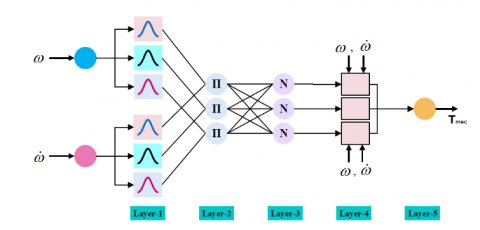Figure 3. Two-Input Sugeno Type ANFIS Structure

This research ANFIS structure is a neural network (NN) which is trained at the beginning to established IF-THEN fuzzy rules and define membership functions for input and output test system parameters. This structure is basically a multi-layer NN and it learns using the back-propagation algorithm. To demonstrate how the ANFIS system works, when a training input-output example is presented to the system, the back-propagation algorithm calculates the output of the system and compares it with the preferred output of the training example. The error is transmitted backwards over the network from the output layer to the input layer. The neuron activation functions are adjusted as the error is transmitted. The diagram assembly of the proposed controller that is engaged in the control algorithm is presented in Figure 3.

3.2.2 ANFIS controller design

The output of neural i in layer l is can be given by:

$U_{i}^{l}=\sum\limits_{j}^{{{N}_{l-1}}}{w_{ij}^{l}}\times O_{i}^{l-1}+b_{i}^{l}$      (15)

$O_{i}^{l}={{g}^{l}}(U_{i}^{l})$     (16)

l = 1,2: number of layer.

gl activation function of neural of layer l.

We can rewrite the above equations in matrix form as follows:

${{\underline{U}}^{l}}={{W}^{l}}\times {{\underline{O}}^{l-1}}+{{\underline{b}}^{l}}$     (17)

${{\underline{O}}^{l}}={{\underline{g}}^{l}}({{\underline{U}}^{l}})$      (18)

where: ${{\underline{U}}^{l}}={{(U_{1}^{l},U_{2}^{l},.....,U_{N}^{l})}^{T}}$,${{\underline{O}}^{l}}={{(O_{1}^{l},O_{2}^{l},......,O_{N}^{l})}^{T}}$, ${{\underline{b}}^{l}}={{(b_{1}^{l},b_{2}^{l},......,b_{N}^{l})}^{T}}$ and

${{W}^{l}}=\left( \begin{matrix} w_{11}^{l} & w_{12}^{l} & \cdots & w_{1{{N}_{l-1}}}^{l} \\ w_{21}^{l} & w_{22}^{l} & \cdots & w_{2{{N}_{l-1}}}^{l} \\ \vdots & \vdots & \ddots & \vdots \\ w_{{{N}_{l}}1}^{l} & w_{{{N}_{l}}2}^{l} & \cdots & w_{{{N}_{l}}{{N}_{l-1}}}^{l} \\\end{matrix} \right)$

3.2.3 Proposed ANFIS controller implementation

The identification of linear systems is currently a field well mastered by the automation engineer. However, many real processes are complex and have non-linear dynamics. The Figure 4 shows the general diagram of neural identification. In this figure, the neural network is used in parallel with a black box type process to be identified. The error equation $e_{i}=y-\hat{y}$ is used by an appropriate learning algorithm.

## 4.png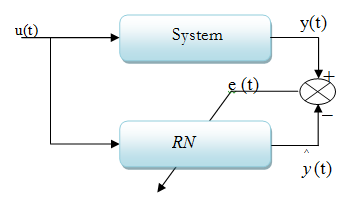Figure 4. Proposed ANFIS controller

The proposed controller structure is modeled in Matlab / Simulink environment to be used in simulation studies as shown in Figure 5.

## 5.png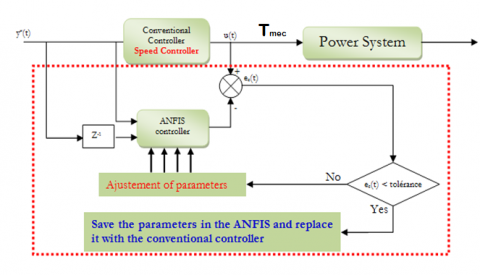Figure 5. Simulation procedure of the proposed controller

In these conditions, the electrical torque is optimized at the optimum operating speeds of synchronous generator shown in Figure 6.

## 6.png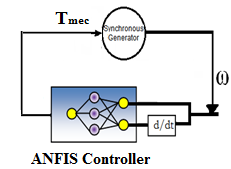Figure 6. ANFIS controller implementation

The inputs are specified for the proposed controller structure. The inputs of ANFIS controller are determined as being the speed of synchronous generator and its derivative. The Gaussian Membership Functions (MFs) are chosen for each input in layer-i which is called as fuzzification layer . The following mathematical expression can be written for Gaussian MF.

$o_{j}^{i}=\exp \left| \frac{{{\left( {{x}_{i}}-{{m}_{ij}} \right)}^{2}}}{2{{\left( {{\sigma }_{ij}} \right)}^{2}}} \right|$      (19)

where, $\sigma$ and $m$ present the standard deviation and mean of MF. In order to update the antecedent and the consequent part parameters through backpropagation algorithm, the squared error (E) which reduces tracking error (e) is expressed as :

$E=\frac{1}{2}{{e}^{2}}$     (20)

If parameter of the proposed controller structure to be adapted is determined as $\vartheta$, the following equation can be used to update the parameter.

$\vartheta (k)=\vartheta (k-1)+\left( -\eta \frac{\partial E(k)}{\partial \vartheta (k)} \right)$    (21)

Here, $\eta$ is the learning rate. The chain rule is employed to get the partial derivative. The derivative chain up to the output of the ANFIS is determine as:

${{\delta }^{1}}=\frac{\partial E}{\partial {{\omega }_{1}}}\frac{\partial {{\omega }_{1}}}{\partial {{T}_{mec}}}\frac{\partial {{T}_{mec}}}{\partial {{y}^{6}}}$    (22)

This controller allows automatic generation of fuzzy rules with five sets based on the SUGENO inference model shown in Figure 7.

## 7.png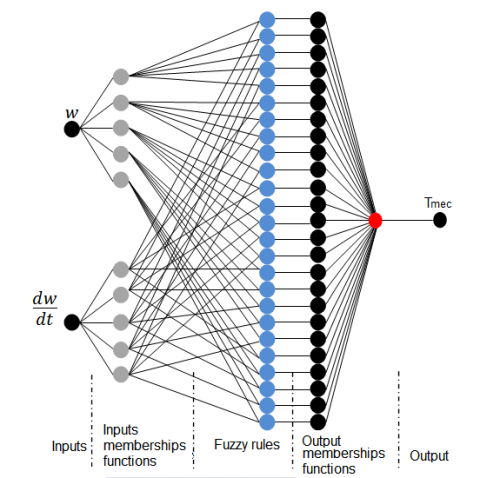Figure 7. Proposed ANFIS controller

## 8a.png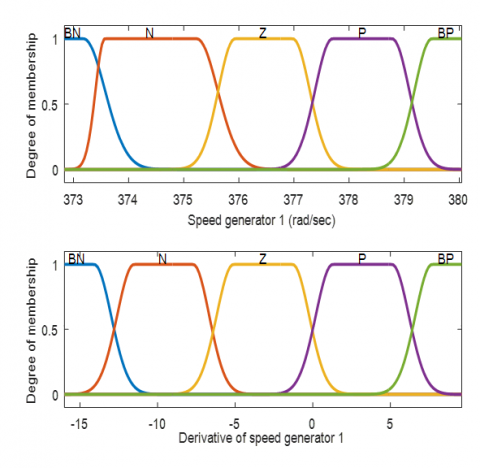## 8b.png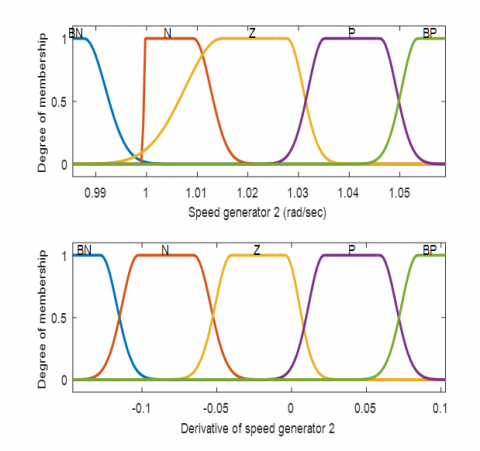## 8c.png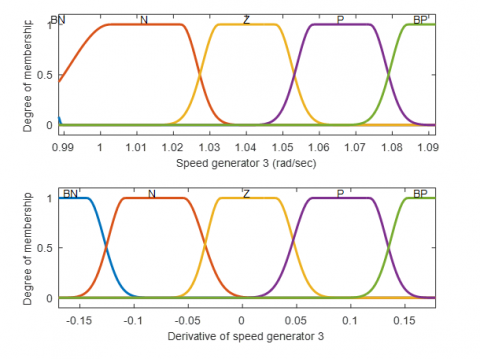Figure 8. Three membership function obtained by ANFIS of 3 machines

In our case, the decentralized controller of three machines which the membership function obtained by ANFIS is given by Figure 8.

4. Time-Domain Simulation Analysis

In order to demonstrate the efficiency, a 3 machines-9 bus system is used to evaluate the proposed model. Time domain simulations using Matlab-Simulink environment are performed for a strong three-phase fault of 0.1 sec applied to bus 4 at line 4-5 shown in Figure 9.

Three distinct scenarios for Test system is considered to demonstrate the ability of the controller to enhance the stability and voltage of the power test system.

The discussions of all the simulation works undertaken in this investigation is described in this section.

## 9.png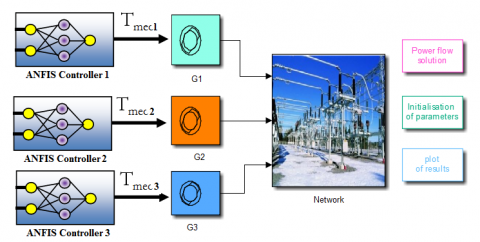Figure 9. Bloc of simulation in Matlab

Scenario 1: A three-phase fault vvin a transmissionvv line between bus 4 and bus 5 at t= 2 s and vvcleared at t = 2.1 s. This scenario without integration of wind farm and without controller.

Scenario 2: A three-phase fault fvvin a transmissionvv line betweenv bus 4 and bus 5 at t= 2 s and vvcleared at t = 2.1 s.. This scenario with integration of wind farm and with conventional regulator

Scenario 3: A three-phasec fault vvin a transmissionvv line between bus 4 and bus 5 at t= 2 s and vvcleared at t = 2.1 s.. This scenario with integration of wind farm and with ANFIS controller.

4.1 Scenario 1

In this scenario, test system is taken as an operating point, because compared with other cases, its having most stable mode. Figure 10 display the open loop eigen values and its damping ratio without integration of wind turbine and without controllers.

It can be noted that the system is transiently stable in these conditions.

4.2 Scenario 2

For this simulation, it is assumed that 3 wind farms are connected in the bus 5, 6 and 8. This wind farm content 21 wind turbine each 1.68MW . In this simulation, the 3 machines are equipped by the conventional regulator. Figure 11 displays the oscillations for the test system after fault. It is obvious from this situation that the system loses its stability. As predicted, the criteria of stability in the presence of wind turbine have a longer value.

## 10a.png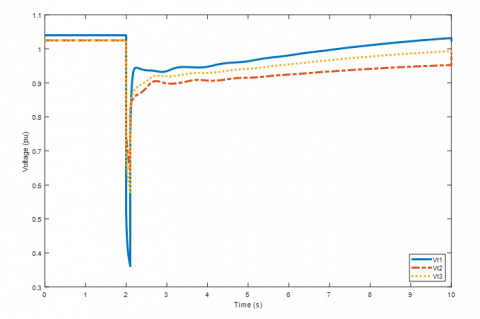## 10b.png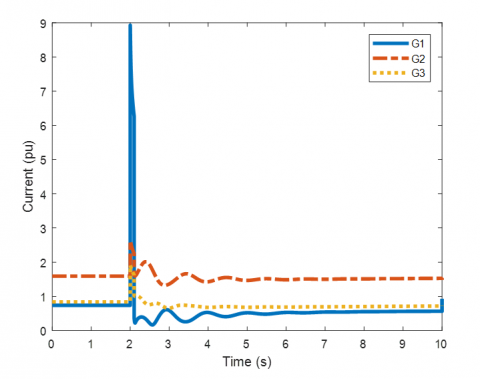## 10c.png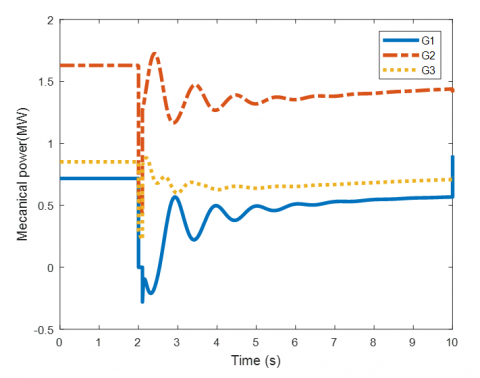## 10d.png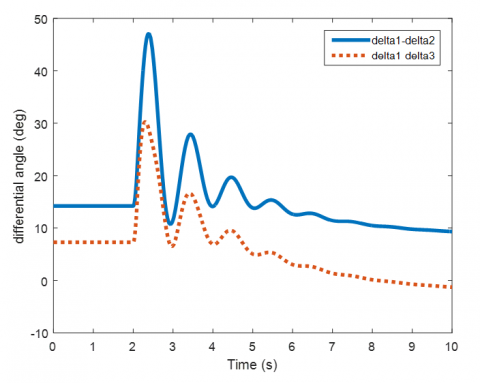Figure 10. Simulation results without wind farm integration and without controller

## 11a.png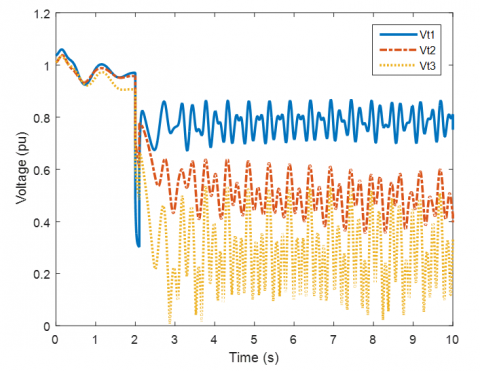## 11b.png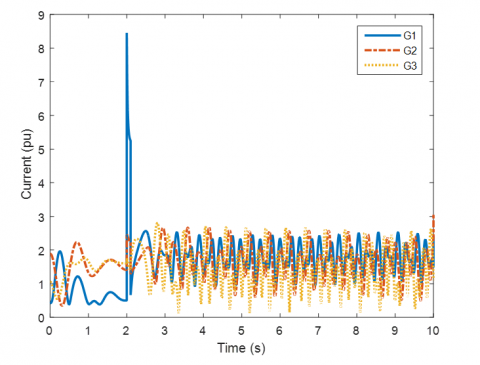## 11c.png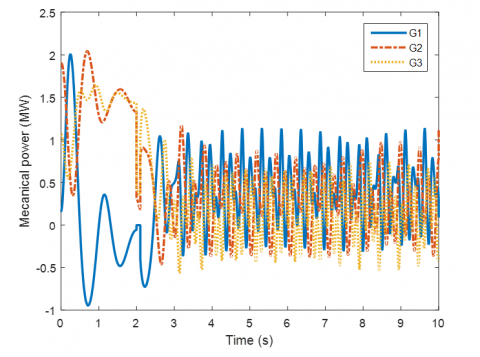## 11d.png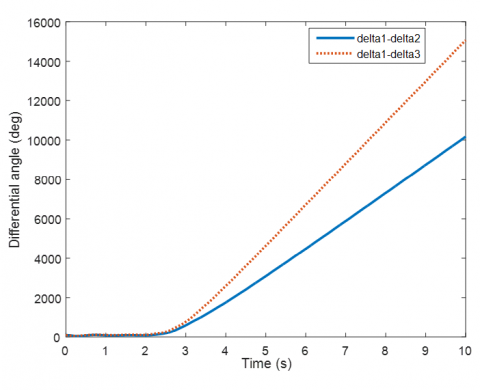Figure 11. Simulation results with wind farm integration and with conventional regulator

4.3 Scenario 3

Also, to examine the reliability of the proposed controller, the proposed ANFIS is applied to large power system and its performances were compared to scenario 1 and 2.

Each Figure 12 includes three plots same as in the test system. The system response with the new controller response reveals that, the dynamic stability of the test system is greatly enhanced using the proposed controller in presence of wind turbine. The proposed controllers fail to give performances containing the minimal number of oscillations. It is noteworthy that, the proposed controller is suitable for large power systems also.

## 12a.png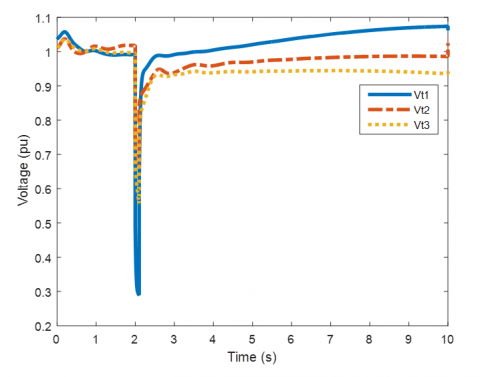## 12b.png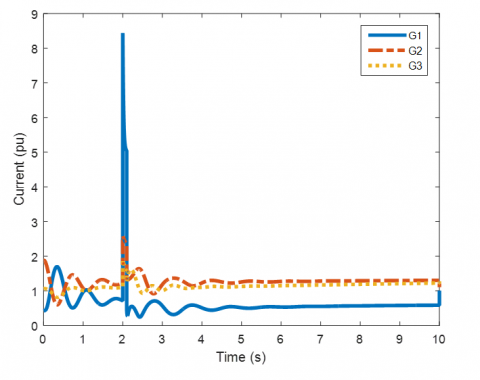## 12c.png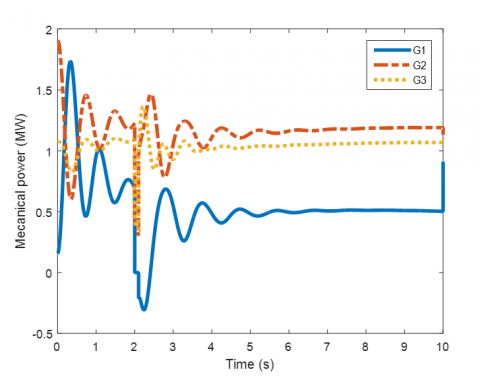## 12d.png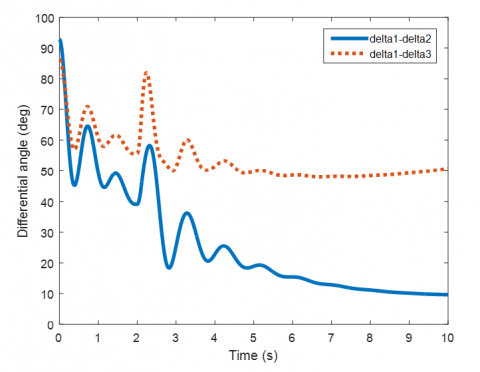Figure 12. Simulation results with wind farm integration and with ANFIS controller

5. Conclusion

In this paper, ANFIS controller has been used to improve the performance of power system in presence of wind turbine. A fair study was conducted on three scenarios: the first without integration of wind farm and without the proposed controller, the second with integration of wind farm and with the conventional regulator and finally with integration of wind farm and with ANFIS controller. The results have been showed that the ANFIS controller capable of improving the dynamical response of power system. The proposed controller was successfully applied to the coordinated design of power system parameters with wind energy generation in order to enhance the voltage of the multi machine power system. The simulations have confirmed the robustness and superiority of the proposed controller for damping characteristics to system oscillations above various types of transient disturbances. These results demonstrate that the proposed controller confirms better disturbance rejection, voltage control, keeps the control quality in the wider operating range and damps the power system oscillations.

Acknowledgements

The authors would like to thank Editors and reviewers for their pertinent comments and suggestions to improve our paper. The authors thank the General Directorate of Scientific Research and Technological Development (DGRSDT).

Nomenclature
 Hi Inertia constant of the i-th generator Di Damping constant of the i-th generator $\omega_{i}$ Rotor angular velocity of the i-th generator $\delta_{i}$ Rotor angle of the i-th generator Pmi Mechanical input power of the i-th generator Pei Electrical input power of the i-th generator $\omega_{b}$ Rotating angular velocity $T_{d 0 i}^{\prime}, T_{q 0 i}^{\prime}$ d-axis and q-axis transient time constants of the i-th generator $E_{d i}^{\prime}, E_{q i}^{\prime}$ d-axis and q-axis transient emf of the i-th generator $x_{d i}^{\prime}, x_{q i}^{\prime}$ d-axis and q-axis transient reactances of the i-th generator $I_{d 0 i}^{\prime}, I_{q 0 i}^{\prime}$ d-axis and q-axis of the generator currents of the i-th generator Vex Field voltage controlled Vt Terminal generator voltage KA,KE,KF Gains TA,TE,TF Time constants R Speed regulation of the conventional speed regulator Tc,Ts Time constants Pm(0) Initial mechanical power P1,P2 Intermediate state variables DBt Dead band travel. R Blade radius of the wind turbine Vw Wind stream of velocity $\rho$ Air density Cp Power coefficient of the wind turbine n Number of wind turbine
References

 Bo, Z.Q., Aggarwa, R.K., Johns, A.T., Zhang, B.H., Ge, Y.Z. (1997). New concept in transmission line reclosure using high frequency fault transients. IEE Proceedings -Generation, Transmission and Diwfli, 144(4): 1359-7051. https://doi.org/10.1049/ip-gtd:19971102

 Anderson, P.M., Fouad, A.A. (2003). Power System Control and Stability Piscataway. N.J.: Wiley-Interscience.

 Speidel, S., Braunl, T. (2016). Leaving the grid-The effect of combining home energy storage with renewable energy generation. Renew Sustainable Energy Rev., 60: 1213-1224. https://doi.org/10.1016/j.rser.2015.12.325

 Hammons, T.J. (2008) Integrating renewable energy sources into European grids. Int. J. Electr. Power Energy Syst., 30: 462-475. https://doi.org/10.1016/j.ijepes.2008.04.010

 Ni, K., Gan, C., Hu, Y., Member, S., Qu, R. (2020). Input-output small-signal stability analysis of A PLLfree direct power controlled partially power decoupled more-electric shipboard propulsion system. IEEE Trans. Transp. Electrif., 7782: 1-15. https://doi.org/10.1109/TTE.2020.3044884

 Li, Y., Shuai, Z., Fang, J., Wu, X., John Shen, Z. (2020). Small-signal stability analysis method for hybrid AC-DC systems with multiple DC buses. IEEE J. Emerg. Sel. Top. Circuits Syst., 3357(c): 1-11. https://doi.org/10.1109/JETCAS.2020.3044091

 Li, H.Z., Guo, S., Li, C.J., Sun, J.Q. (2013). A hybrid annual power load forecasting model based on generalized regression neural network with fruit fly optimization algorithm. Knowledge-Based Systems, 37: 378-387. https://doi.org/10.1016/j.knosys.2012.08.015

 Ganjefar, S., Alizadeh, M. (2012). Inter-area oscillations damping by multi-objective wavelet neural inverse controlled SSSC. International Review of Electrical Engineering, 7(2): 281-290.

 Saidi, S., Abbassi, R., Chebbi, S. (2015). Fuzzy logic controller for three level shunt active filter compensating harmonics and reactive power. International Journal of Adaptive Control and Signal Processing, 45: 320-329. https://doi.org/10.1016/j.epsr.2009.04.003

 Alizadeh, M., Tofighi, M. (2013). Full-adaptive THEN-part equipped fuzzy wavelet neural controller design of FACTS devices to suppress inter-area oscillations. Neuro Computing, 118: 157-170. https://doi.org/10.1016/j.neucom.2013.03.001

 Ghadimi, N. (2015). A new hybrid algorithm based on optimal fuzzy controller in multi-machine power system. Complexity, 21(1): 78-93. https://doi.org/10.1002/cplx.21544

 Tvakoli, A.R., Seifi, A.R., Arefi, M.M. (2016). Fuzzy- PSS and fuzzy neural network non-linear PI controller based SSSC for damping inter-area oscillations. Trans. Inst. Meas. Control, 40(3): 733-745. https://doi.org/10.1177%2F0142331216665688

 Domínguez-García, J.L., Gomis-Bellmunt, O., Bianchi, F.D., Sumper, A. (2012). Power oscillation damping supported by wind power: A review. Renewable and Sustainable Energy Reviews, 16(7): 4994-5006. https://doi.org/10.1016/j.rser.2012.03.063

 Mahmud, N., Zahedi, A. (2016). Review of control strategies for voltage regulation of the smart distribution network with high penetration of renewable distributed generation. Renew Sustainable Energy Rev., 64: 582-595. https://doi.org/10.1016/j.rser.2016.06.030

 Wu, Y.K., Han, G.Y., Lee, C.Y. (2013). Planning ten onshore wind farms with corresponding interconnection network and power system analysis for low-carbon-island development on Penghu Island, Taiwan. Renewable Sustainable Energy Rev., 19: 531-540. https://doi.org/10.1016/j.rser.2012.10.043

 Slotine, J.J.E., Li, W.P. (1991). Applied Nonlinear Control. Prentice-Hall, Englewood Cliffs, NJ.

 Griche, I., Messalti, S., Saoudi, K., Touafek, M. (2019). A new adaptive neuro-fuzzy inference system (ANFIS) and PI controller to voltage regulation of power system equipped by wind turbine. European Journal of Electrical Engineering (EJEE), 21(2): 149-155. https://doi.org/10.18280/ejee.210204

 Griche, I., Messalti, S., Saoudi, K. (2019). Parallel fuzzy logic and pi controller for transient stability and voltage regulation of power system including wind turbine. Przegląd Elektrotechniczny. https://doi:10.15199/48.2019.09.10

 Çötelİ, R., Açikgöz, H., Dandil, B., Tuncer, S. (2018). Real-time implementation of three-level inverter-based D-STATCOM using neuro27 fuzzy controller. Turkish J. Electr. Eng. Comput. Sci., 26: 2088-2103. https://doi.org/10.3906/elk-1708-281

 Li, X., Niu, Y., Fu, J., Zhang, X., Lin, Z., Niu, Y.G. (2018). A neural power system stabilizer of DFIGs for power system stability support. Int. Trans. Electr. Energy Syst., 28(6): 1-20. https://doi.org/10.1002/etep.2547

 Muljono, A.B., Ginarsa, I.M., Nrartha, I.M.A., Dharma, (2018). Coordination of adaptive neuro fuzzy inference system (ANFIS) and Type-2 fuzzy logic system-power system stabilizer (T2FLS-PSS) to improve a large-scale power system stability. Int. J. Electr. Comput. Eng., 8(1): 76-86. http://doi.org/10.11591/ijece.v8i1.pp76-86

 Wang, C., Gao, Q., Hou, Y.L., Min, H. (2016). Adaptive complementary fuzzy self-recurrent wavelet neural network controller for the electric load simulator system. Trans. Inst. Meas. Control, 8(3): 733-745. https://doi.org/10.11772F1687814016639250

 Sambariya, D.K., Prasad, R. (2017). A novel fuzzy rule matrix design for fuzzy logic-based power system stabilizer. Electr. Power Components Syst., 45(1): 34-48. https://doi.org/10.1080/15325008.2016.1234008

 Kundur, P. (1994). Power System Stability and Control. New York: McGraw Hill.

 Namjoo, N., Abbasi, F., Hassanzadeh, F., Asrari, H. Hajizadeh, A. (2014). A new hybrid control method for controlling back-to-back converter in permanent magnet synchronous generator wind turbines. J. Renewable Sustainable Energy, 6: 133-142. https://doi.org/10.1063/1.4884198

 Kececioglu, O.F., Acikgoz, H., Yildiz, C., Gani, A. (2017). Power quality improvement using hybrid passive filter configuration for wind energy systems. J Electr Eng Technol, 12(1): 207-216. https://doi.org/10.5370/JEET.2017.12.1.207

 Acikgoz, H., Kececioglu, O.F., Gani, A., Yildiz, C., Sekkeli, M. (2016). Improved control configuration of PWM rectifiers based on neuro - fuzzy controller. Springer Plus, 5(1142): 1-19. https://doi.org/10.1186/s40064-016-2781-5